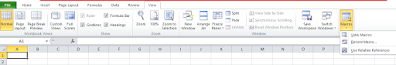Whether in you are in any field like sales or education & etc. You can not escape from Microsoft’s Excel as it is necessary nowadays with so many functions almost anybody can find a use for this. But at the same time, Few got mastered it. While the sheer capacity of the application can be scary, no uncertainty of realizing how to utilize Excel gives any understudy an edge in the working environment. Here we have some excellent tips.Microsoft Excel Tricks and Functions

## Essential Microsoft Excel Tips

1). Vlookup

2). Filters

3). Sum

4). Sumif

5). Count

6). CountA

7). Average

8). PivotTable

9). Dashboard in Excel

10). Data Validation

11). Picture Lookup

12). Hlookup

13). Data transfer from excel to word

14). Data transfer from notepad to excel

We can see a few functions above as mentioned as essential functions or formulas. Microsoft Excel has many features or functions to use however what functions are considered basic is the question here. Because first of all, we need to learn basic functions and then we can go for advanced-level excel to become a masters in excel.

Basic Functions are those functions that we use easily for basic work in excel like the sum and average, etc. These are just examples. It does not mean that only these are the basic functions, There are as many as functions considered basic like vlookup,  hlookup and many more.

As we have seen other few names as well above in the list to learn essential Microsoft excel tips, there are also a few functions we saw as advanced level excel too like creating a dashboard in excel, picture lookup, etc.

Now here will discuss the basic and advanced level functions however once we get to know about the features of basic and advanced then also we can call ourselves the master in excel.

### The formula will be = Vlookup (lookup value, table array, column number,(range lookup)). The screenshot is attached here for a better explained below.Microsoft Excel tricks and functions

#### Filters

Filters are the best options to look at any specific data within a large data set, You can reduce the data to check certain criteria (without changing original data)

For example:- I might want to look at the subjects only from particular states.Microsoft Excel tricks and functions

Now you can check this screenshot above, Filters have taken their places now you may have to click the filters and select the heads or subheads to check and all will be easily shown as done.

Basically what we are discussing here is that if we learn basic and advanced-level functions in excel then, of course, we can even learn new tricks in excel.

Now we will add a few functions here in the excel tricks with examples. First of all let s discuss the most important feature of excel, called MACRO.

Question:- What is macro in excel?

Answer:- When we have a task to repeat any data work that we need to make as repeatedly then we can simply record a macro for it. Macro saves time and we can run it as much as time. That is called macro.

Question:- How to record a macro in the excel sheet?

Answer:- Let's suppose we have to create data in the excel sheet where we need to perform repetitive work means there is work that we do that is coming under repetition. Now here we will record a macro so that repetition will be stopped and it will create the data itself easily. Let c how it works.

Now we are recording a macro so we need to open our excel sheet and if it is Microsoft Office 2010 then look at the last button on the top called VIEW and click on it. Once we click on it then we have many options but we need to focus on the last options as says macro. Check the screenshot below.Recording a macro

As per this image check in the last that we have 3 options altogether then we have to see all of them and us going to click the first one from the bottom.

1). View Macro

2). Record Macro

3). Use relative references

Now let us get the details of View Macro first.

1). View Macro – If we already recorded a macro in the excel sheet then we click this VIEW MACRO to run or view the macro in the spreadsheet.

It also has many features when we click it.

### Like As:-

1)Run – This option runs the macro.

2)Edit- It gives a chance again to edit the macro coding.

3)create – Here we create the macro.

4)Delete- We can delete the macro in the excel sheet by clicking it.

5)Options – Here we get many other functions that we can check here in the screenshot below.

2). Record Macro – Here we record a macro and also we have the option to create a shortcut for running it as if like [CTRL+SHIFT+()]. Remember when we create a shortcut then we also press a shift key if we do so then we will not be confused about the shortcut key to run the macro.

Even if we forget the shortcut key then we can simply run it by clicking the first option that we have in the top VIEWMACRO, Here we get a button as RUN to run the macro.

For better understanding let us watch the video here for recording a macro.